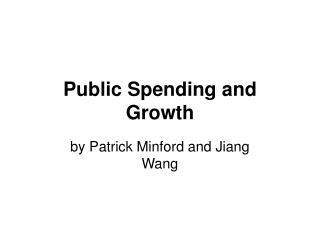DownloadDownload PresentationPublic Spending and Growth

# Public Spending and Growth

Download Presentation## Public Spending and Growth

- - - - - - - - - - - - - - - - - - - - - - - - - - - E N D - - - - - - - - - - - - - - - - - - - - - - - - - - -
##### Presentation Transcript

1. Public Spending and Growth by Patrick Minford and Jiang Wang

2. TWO VIEWS • Endogenous growth, public spending and taxes: two views • R&D, education and infrastructure spending by the state creates new factor input; hence growth. ‘ACTIVIST’ • Incentives generate risk-taking innovation by people/firms. Lower taxes raise incentives; hence growth. ‘INCENTIVIST’

3. ECONOMETRIC TESTS • INCENTIVIST: Growth negatively related to the marginal tax rate on innovation. • ACTIVIST: Growth positively related to state support of R&D and subsidy of the return on capital. • Data: growth rate over three decades (1970s, 1980s, and 1990s) for 100 countries; 300 panel observations (some missing).

4. Below each parameter estimate, we report its robust standard error. Three asterisks denote statistical significance at the 1% level, two asterisks at the 5% level and one asterisk at the 10% level.lnGadj = ln(G+0.04) due to the possible negative value of G for some countries. Observations with growth less than –0.04 (-4% per annum) are omitted as outliers with special reasons (such as civil wars etc) Table 1

5. Figure1: Correlation between growth rate in real GDP per capita and tax rate

6. Table 2: Initial value of GDP per capita ― lnG0

7. Table 3:Initial value of human capital variable ― lnhc0

8. Table 5: Ratio of investment/GDP ― lninvest

9. Table 6: Adding lnG0, lnhc0, lnpc0 and lninvest

10. Table 7: Results for the activist approach

11. Figure 2 Correlation between GDP growth rate and subsidy rate to investment

12. Table 8: Initial value of GDP per capita ― G0

13. Table 9: Initial value of human capital variable ― hc0

14. Table 10: Initial value of physical capital variable ― pc0

15. Table 11: Ratio of investment/GDP ― invest

16. Table 12: Adding G0, hc0, pc0 and invest

17. CONCLUSIONS • Tax elasticity of -0.4; meaning for initial tax rate of 40% and growth rate of 2.5%. Fall in marginal tax of 10% (ie to 36%) raises growth by 0.1% to 2.6%. • No evidence in this data of effects of state spending on R&D or on subsidy to investment. • Further work on structural models required.